Function Repository Resource:

# ConditionalProductDistribution

Represent a product of distributions where some distributions can depend on others

Contributed by: Sjoerd Smit
 ResourceFunction["ConditionalProductDistribution"][Distributed[var1,dist1],Distributed[var2,dist2],…] represents the multivariate distribution of {var1,var2, …} where each disti may depend on varj for all i

## Details

ResourceFunction["ConditionalProductDistribution"] is used to define a multivariate distribution in terms of conditional probabilities using the conditional product rule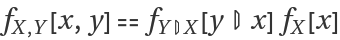(where f𝒟 denotes the PDF of a distribution 𝒟).
ResourceFunction["ConditionalProductDistribution"] is like a hybrid between ProductDistribution (which allows you to draw from multiple independent distributions at once) and ParameterMixtureDistribution (which allows you to define new distributions in terms of existing ones).
ResourceFunction["ConditionalProductDistribution"] works with RandomVariate, PDF, Likelihood and LogLikelihood and Mean. It does not necessarily work in all Wolfram Language functions for distributions.
Graph can be used to create a dependency graph of the variables vari.
ResourceFunction["ConditionalProductDistribution"] will return \$Failed if one of the disti depends on some varj for ij.

## Examples

### Basic Examples (2)

Define a BinomialDistribution where the probability of success is drawn from a BetaDistribution:

 In:=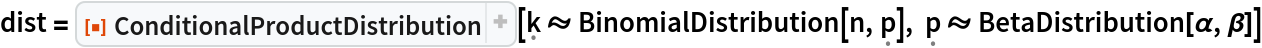Out=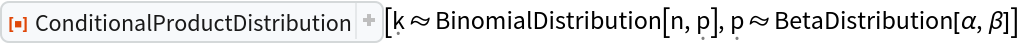Compute the PDF:

 In:=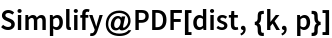Out=Compute the Mean:

 In:=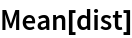Out=Draw random samples from the distribution. Each sample returns the values of k and p:

 In:=Out=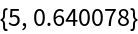Generate multiple samples:

 In:=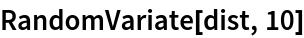Out=Calculate the LogLikelihood of the samples:

 In:=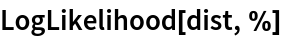Out=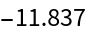Samples with lower values of k are generally produced by samples with lower values of p:

 In:=Out=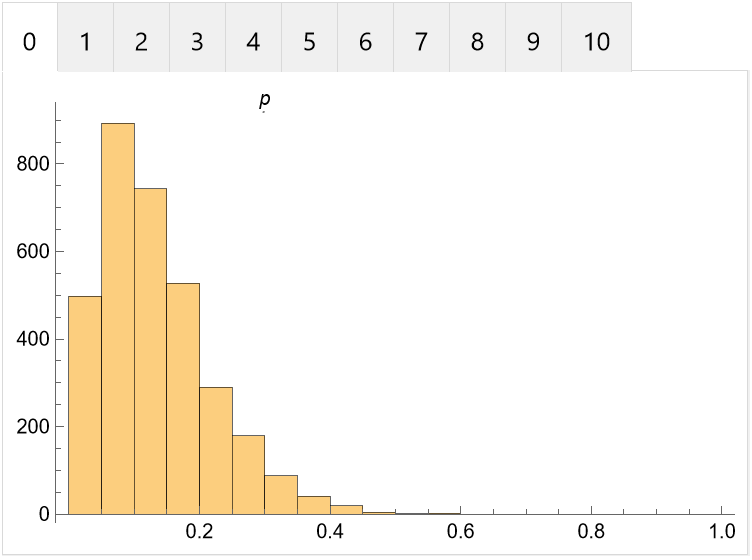### Scope (2)

Graph can be used to generate a dependency graph of the individual random variables. Each edge x y should be read as "x influences y":

 In:=Out=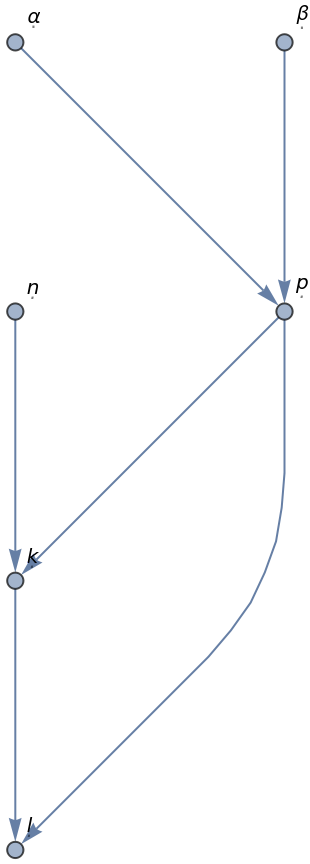The graph is acyclic:

 In:=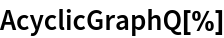Out=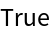Sample the distribution and compute the expected value. Note that the coordinates are listed in order of appearance of the symbols:

 In:=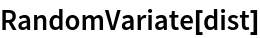Out=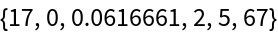In:=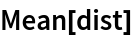Out=In:=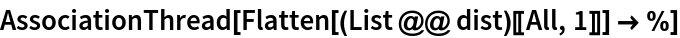Out=When a ConditionalProductDistribution is defined using unprotected symbols for the bound variables, they will be replaced with symbols of the form x[i]:

 In:=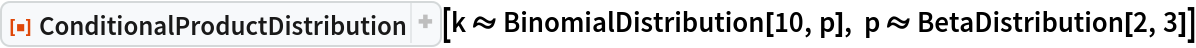Out=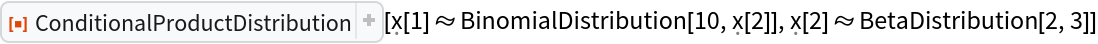### Applications (3)

Define a Normal-inverse-Wishart distribution, which is the conjugate prior to a multivariate normal distribution of unknown mean and covariance:

 In:=Draw samples from it:

 In:=Out=Draw samples from the posterior predictive distribution:

 In:=Out=### Properties and Relations (3)

A ParameterMixtureDistribution is the marginal distribution of a ConditionalProductDistribution:

 In:=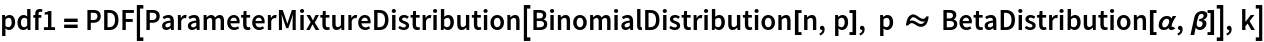Out=Compute the marginal over k by integrating out p from the PDF of the corresponding ConditionalProductDistribution:

 In:=Out=The result is the same:

 In:=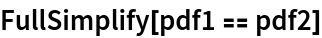Out=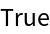### Possible Issues (3)

The distributions need to be ordered correctly and cannot be circular:

 In:=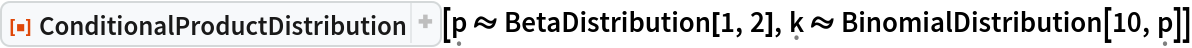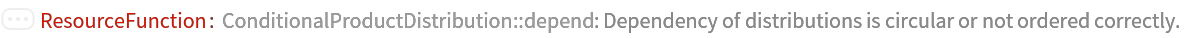Out=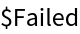In:=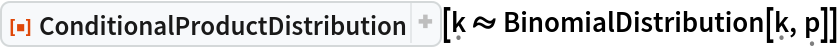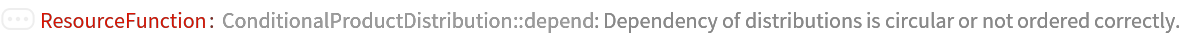Out=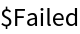In some cases PDF cannot distinguish between evaluating a single coordinate or threading over multiple coordinates. In the following example, the second argument will be interpreted as a pair of coordinates rather than a single 2×2 coordinate:

 In:=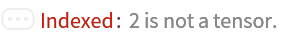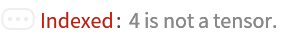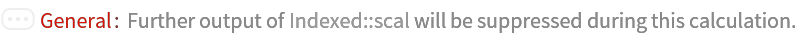Out=In this case, wrap the second argument in a list to remove the ambiguity:

 In:=Out=Bound variables should not have definitions at the time of defining a ConditionalProductDistribution, since this can have unexpected results:

 In:=Out=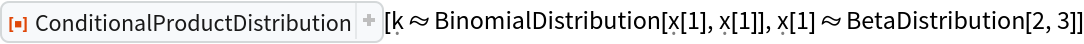In:=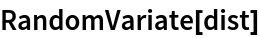Out=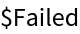Use Clear or Block to make sure p has no value at the moment of definition. After that, the variable will have been localized so that p can be used again:

 In:=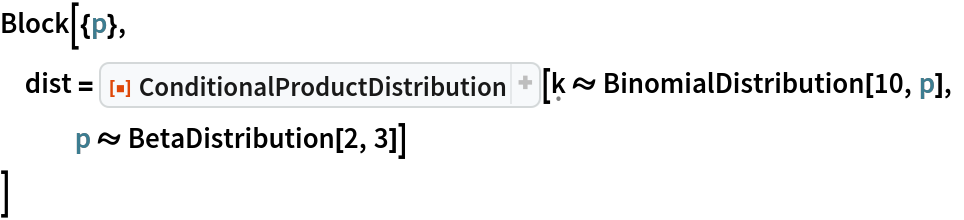Out=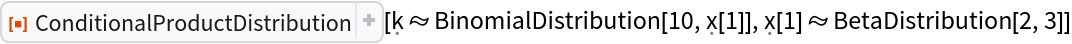In:=Out=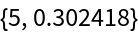### Neat Examples (3)

Define a function that can compute the Expectation of a ConditionalProductDistribution:

 In:=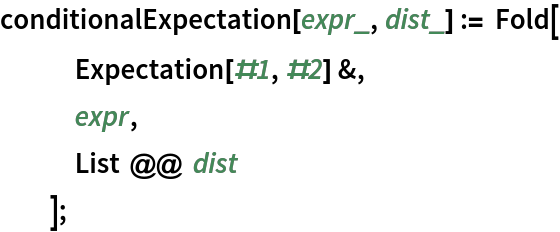Define a distribution using formal symbols (to ensure they do not get renamed) and compute an expected value:

 In:=Out=Check the result with a Monte Carlo simulation:

 In:=Out=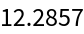In:=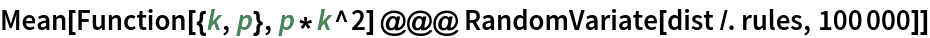Out=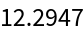Sjoerd Smit

## Version History

• 1.1.0 – 10 February 2023
• 1.0.0 – 14 August 2020